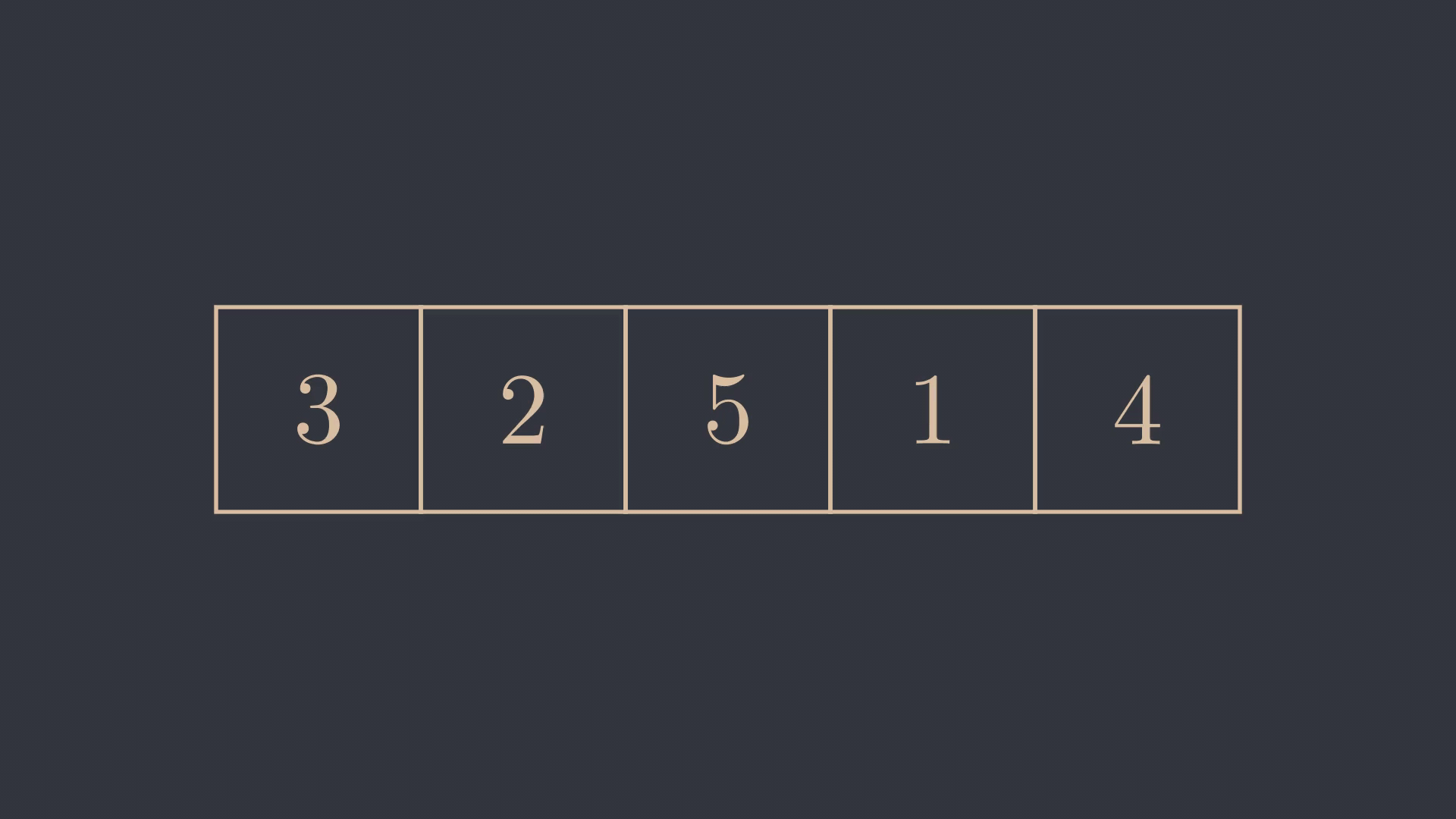# The Bubble Sort Algorithm

18 February 2022

Motivation

Bubble Sort is usually the first algorithm that beginners encounter when learning to sort. Although Bubble Sort is not an efficient algorithm, it still plays an important role in the literature and appears in most textbooks on algorithms and computer science. I believe this is the case because Bubble Sort is possibly the most elementary and natural sorting algorithm there is. If you were to ask a beginner to design a sorting algorithm, the result would probably be Bubble Sort.

How does it work ?

The idea behind the algorithm is really simple. Suppose we want to sort a list of numbers in ascending order, then we start by going through all the consecutive pairs of elements. If the first value of the pair is greater than the second, we swap both elements so that the largest element "bubbles up" to the top.As we can see in the animation above, after iterating through the list and swapping the corresponding elements, we still do not have a sorted list, but if you look closely, the largest element always makes the swap until it reaches the end. All we have to do now is repeat this process for the remaining elements. Each time we repeat this, the next largest element will be swapped until it reaches the correct position.Implementation

Now that we have seen how Bubble Sort works, let's see how it might be implemented. The outer loop repeats the process $$N$$ times to make sure all elements are ordered, while the inner loop goes through all pairs and swaps them if the condition is met.

void BubbleSort (int n, int a[]) {
for (int i = 0; i < n; i++) {
for (int j = 0; j < n - i - 1; j++) {
if (a[j] > a[j + 1]){
swap(a[j], a[j + 1]);
}
}
}
}


Note that the boundary of the inner loop is bounded by the iteration of the outer loop. We have already seen that a suffix made of ordered elements will start to form, hence we don't need to check if those are in the correct order.

Swap condition

We assumed that we wanted to sort an array of integers in ascending order. But what if we want to sort in a different order, or using different data types like strings? To do so is quite simple, we just need to change the swap condition in the if statement. If we want to sort strings by their length, we can simply use the following condition.

if (a[j].length > a[j + 1].length){
swap(a[j], a[j + 1]);
}


Asymptotic Analysis

As mentioned earlier, the bubble sort algorithm is inneficient. This is mainly due to the nested loops, which indicate a quadratic time complexity. Let us perform a more detailed analysis to draw our own conclusions. In the first iteration of the outer loop, the inner loop performs $$N-1$$ iterations, in the second $$N-2$$, and so on until it reaches $$0$$, therefore, in the worst case, the algorithm performs the following number of operations:
$$(N-1) + (N-2) + ... + 2 + 1$$ Since this is an arithmetic progression, it is translated in this expression: $$\frac{N(N-1)}{2} = \frac{N^2 - N}{2}$$ From this we conclude that the time complexity must be $$O(N^2)$$. This is nowhere good since most decent sorting algorithms run in $$O(N\log(N))$$, and even among the other sorting algorithms with quadratic time, Bubble Sort performs worse. This does not mean that the algorithm is useless. If you need to sort a list of items and efficiency is not an issue, Bubble Sort is quite simple and quick to implement.